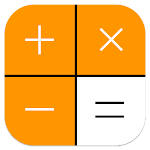ID: com.entiseyinapps.calaculatorapps212e

• Author:

• Version:

13

• Update on:

The description of Scientific calculator

Scientific calculator 300 es plus, 991 ex
Is a scientific calculator for students of engineering. The 991 es Plus calculator provides
Powerful functions in real calculator 991 ex 300 ex.
Makes the advanced calculator 991 ex
The scientific calculator becomesthe most useful for the University and school.

The application has some calculators inside:
- Basic calculator 300 es plus
- Advanced calculator 115 es plus
- Scientific calculator 991 ex
- Scientific calculator 991 es plus
- Graphic calculator 84 plus

Let's see some of the special features:

calc basic calculator 300 es plus and 115 es plus: supports from basic math to advanced math functions: percentage, powers, roots, trigonometry, logarithm calculator. Fraction calculation 991 ex supports converting fraction to decimal and mixed fraction and solving fractional problems.

◉ Advanced calculator 115 es plus: combines hyper calculator with simple scientific calculation. This calculator is an advanced multi-function 991 ex calculator with all features in one app. Like linear algebra, calculus, complex numbers, the display produces rectangular and polar coordinates, Matrix and Vector.

◉ Scientific calculator 991 es plus: includes equation solving smart. Equation solver can solve quadratic, cubic equations and systems of equations. Caluculator solves any polynomials. The smart scientific calculator 991 ex has an exponential calculator that contains scientific calculations such as derivatives, integrals, square root calculation, factor calculation, pi calculation and solution equation.

ulator scientific calculator 991 ex: supports calculation with infinite number of numbers, prime factors, random numbers, combinations, relays, GCD and LCM. This 991ex calculator is a real engineering simulator. The scientific calculator has features of 500 es, 500 ms, 300 es plus, 991 es plus. The layout of the calculator keyboard is the same as the calculator 300 es plus, 991 ex and es plus.

calc 991 es Plus integrated calculator provides a powerful calculator for calculating integration, derivatives, differentiation and integration.

◉ This scientific calculator includes 991ex CAS (computer algebra system), which can perform a symbolic calculation.

◉ Some other features: math formula, physics formula, unit conversion, attribute, font and programming.